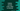# Python program to convert a hexadecimal value to decimal## Python program to convert a hexadecimal value to decimal:

In this post, we will learn how to convert a hexadecimal value to decimal in Python. As we know that hexadecimal number system is a base-16 number system and decimal number system is a base-10 number system. In both number system, we use different ways to represent a number.

In hexadecimal, 0 to 9 and A to F are used to represent a number. Instead of A to F, we can also use a to f, i.e. in lower case. But, in decimal number system, 0 to 9 is used to represent a number.

We need to follow a specific algorithm to do the conversion.

### Method 1: Standard way:

The standard way to convert a hexadecimal value to decimal is by multiplying each digit of the number by 16 to the power of its index position.

So, we need to start from the rightmost digit of the hexadecimal number. We can consider its position as 0, the second rightmost digit as 1 etc. For the rightmost digit, we will multiply it by 16^0, for the second rightmost digit, we will multiply it by 16^1 etc. And finally we will add all of these values to find the decimal value.

But, hexadecimal numbers also includes other non-decimal characters i.e. A, B, C, D, E, F or a, b, c, d, e,f. So, we have to use the following mapping table to get the decimal equivalent to multiply with the 16 power.

hex value decimal value
A or a 10
B or b 11
C or c 12
D or d 13
E or e 14
F or f 15

For other digits 0 to 9, we will use the same value in decimal.

#### Example of hexadecimal to decimal conversion:

Let’s try to convert 2EF4 hexadecimal value to decimal:

``````2 * 16^ 3 + 14 * 16^2 + 15 * 16^1 + 4 * 16^0
= 12020``````

As you can see here, we are multiplying each digit with 16 to the power of its position.

### Python program:

Below is the complete python program that takes a hexadecimal value as input from the user and prints the decimal value for it:

``````def hex_to_decimal(hex):
hex_decimal_conversion = {'0': 0, '1': 1, '2': 2, '3': 3, '4': 4, '5': 5, '6': 6, '7': 7, '8': 8, '9': 9,
'A': 10, 'a': 10, 'B': 11, 'b': 11, 'C': 12, 'c': 12, 'D': 13, 'd': 13, 'E': 14, 'e': 14, 'F': 15, 'f': 15}

p = len(hex) - 1
decimal = 0

for c in hex:
decimal += hex_decimal_conversion[c] * (16 ** p)
p -= 1

return decimal

hex = input('Enter the hexadecimal value: ')
decimal = hex_to_decimal(hex)

print(f'Decimal of {hex} is {decimal}')``````

In this program,

• hex_to_decimal method is used to convert a hexadecimal string to decimal value. It takes the hexadecimal string as its parameter and returns the decimal value.
• hex_decimal_conversion is a dictionary with keys are hexadecimal values and values are decimal representation. It has the mapping for both uppercase and lowercase hexadecimal characters.
• p is the power to use for 16
• decimal variable is initialized as 0 to hold the final decimal value.
• The for loop iterates throught the characters of the hexadecimal string one by one. For each character, it uses the dictionary to get the decimal value and multiply it with 16 to the power p. It adds that value to decimal.
• It decrements the value of p by 1 before moving to the next digit.
• Once the loop ends, it returns the decimal value calculated.

If you run this program, it will print output as like below:

``````Enter the hexadecimal value: EF
Decimal of EF is 239

Decimal of 2ef4 is 12020``````

As you can see here, it works with both uppercase and lowercase characters.

### Method 2: By using int():

This is the easiest way to convert a hexadecimal to decimal in python. We can simply pass the hexadecimal value to this method and it will return us the converted decimal value.

Hexadecimal numbers are prefixed with 0x or 0X. So, if we are passing any hexadecimal number that starts with 0x or 0X, int() will automatically detect that it is a hexadecimal value and it will convert it to decimal.

But, if we are passing string to int(), then we have to pass another second parameter as 16 to define that the conversion is for hexadecimal values. It will convert that string to decimal and return the decimal value.

``````print(f'0XEF => {int(0XEF)}')
print(f'0xef => {int(0xef)}')

print(f"2ef4 => {int('2ef4', 16)}")
print(f"2EF4 => {int('2EF4', 16)}")``````

If you run this program, it will convert the hexadecimal values to decimal values and print the results.

``````0XEF => 239
0xef => 239
2ef4 => 12020
2EF4 => 12020``````